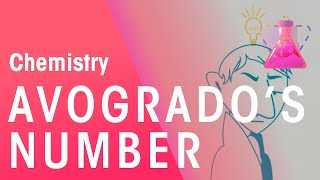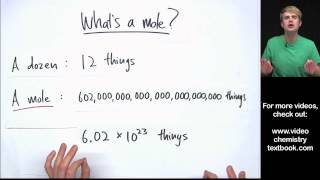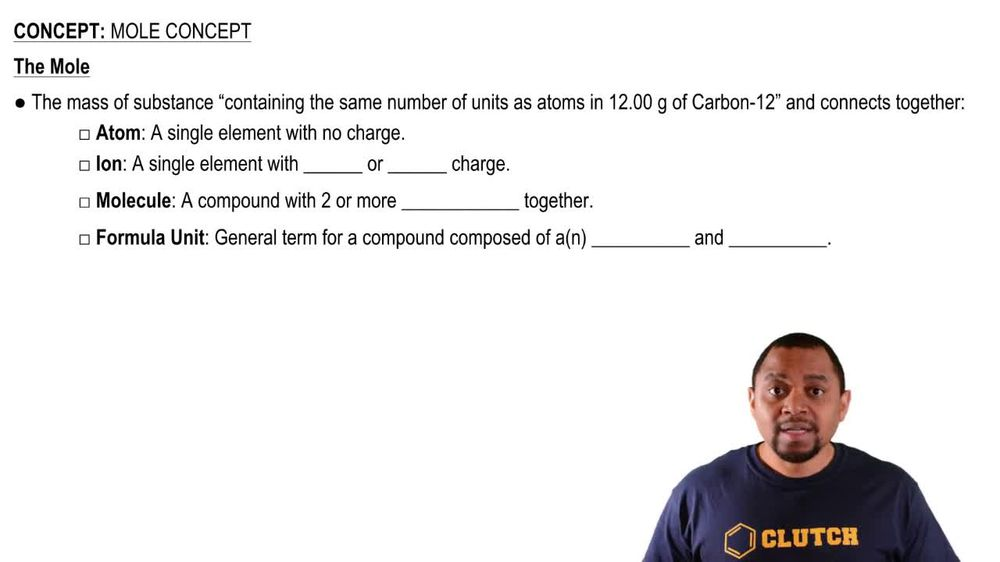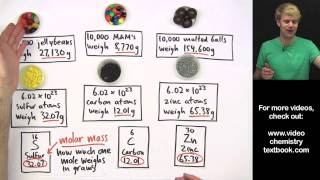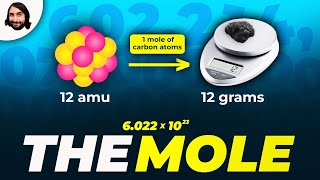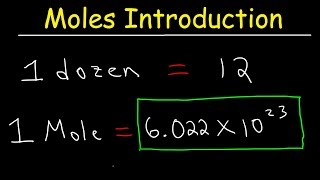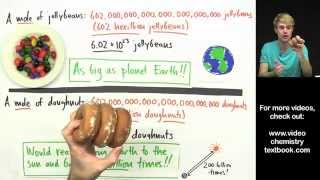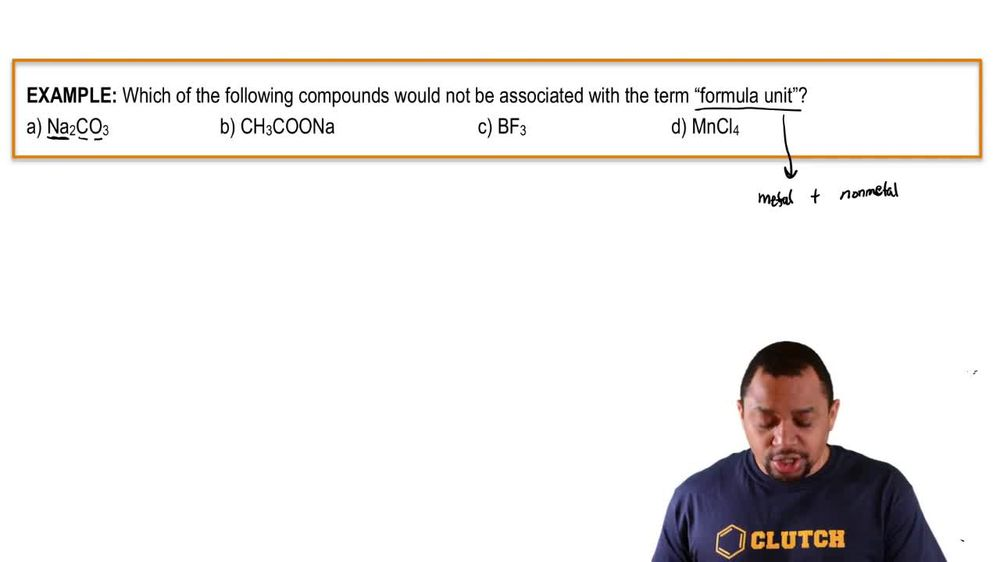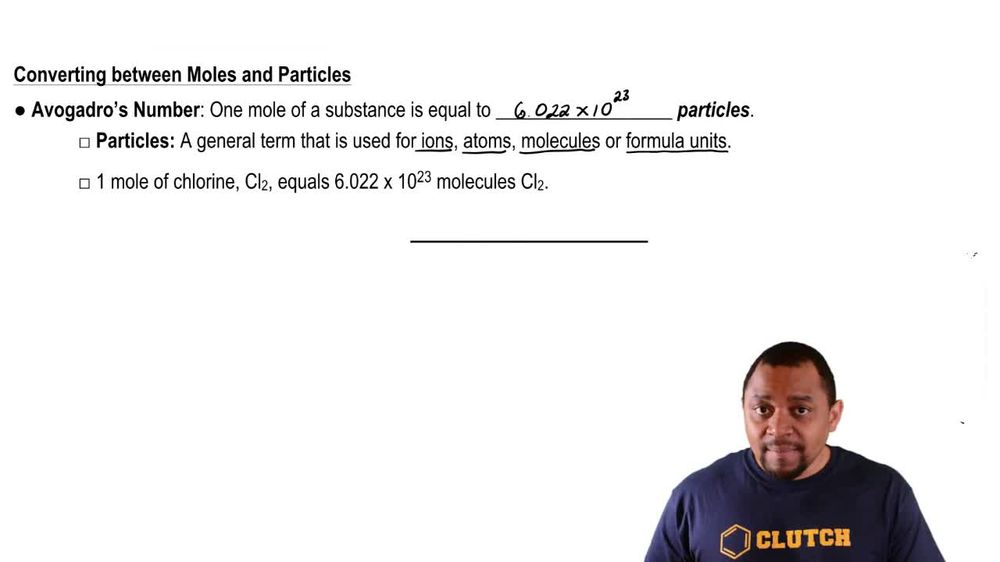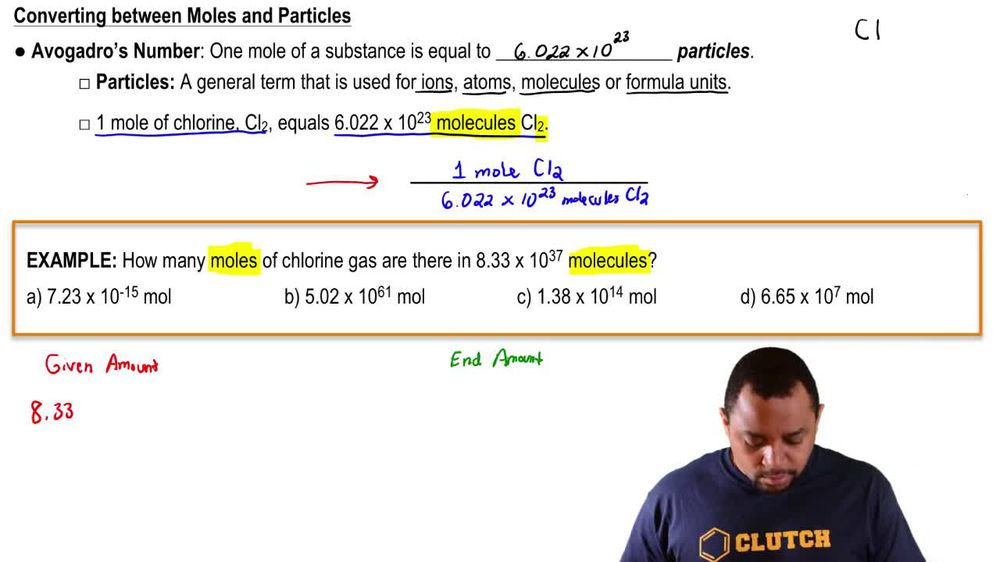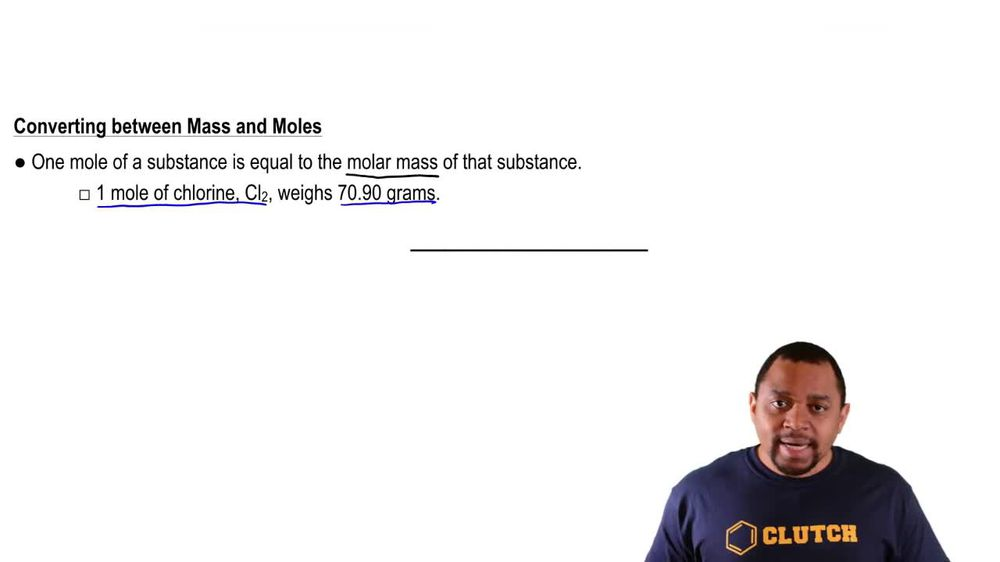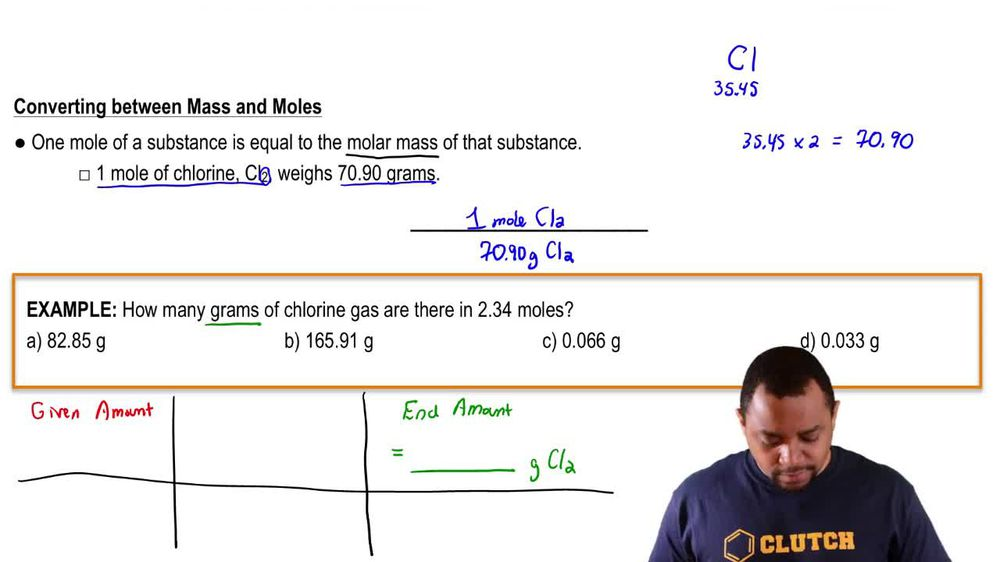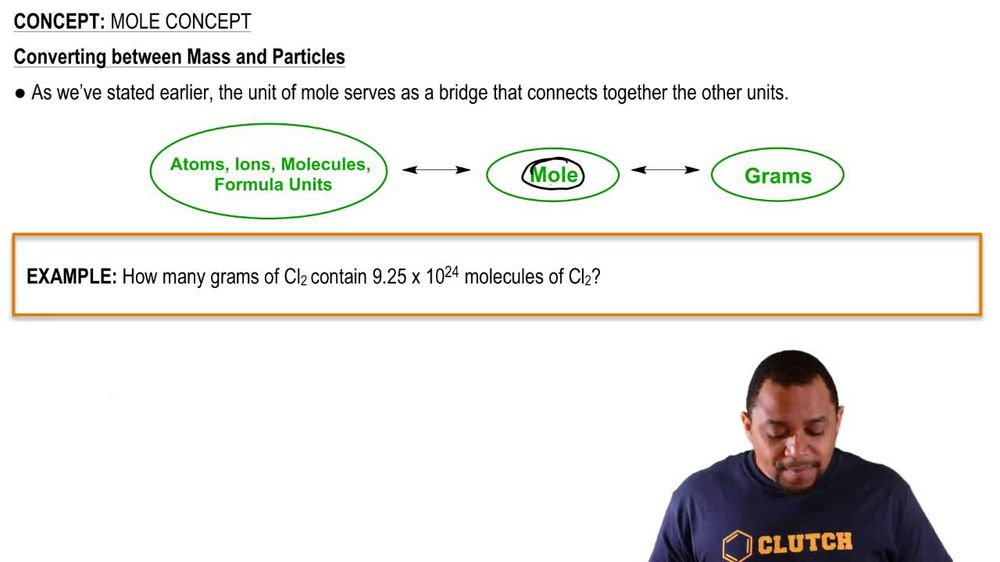Start typing, then use the up and down arrows to select an option from the list.
1. 2. Atoms & Elements2. Mole Concept# Mole Concept Example 2

by Jules Bruno
179 views
1
0
So in this example question, it says how many moles of chlorine gas are an 8.33 times 10 to the 37 molecules? All right, so to be able to do this question, we need to realize that we're trying to go from molecules two moles, right? And to be able to do that, we're gonna have to utilize the conversion factor that we have up here. Alright, so that those molecules are are given amount. Remember, we're setting this up as though it is a dimensional analysis question. Although we're dealing with more concept, it still hinges on the idea of dimensional analysis where we have our given amount and then we need to get to our end amount. And to do that we utilize conversion factors. So if you don't quite remember the steps necessary to do this, go back and take a look on on videos on dimensional analysis. All right, so are giving them out is 8.33 times 10 to the 37 molecules off the L two and we need to get to our end amount, which will be in moles. Moles of cool, too. Now, to be able to go from given amount to end amount, we have to utilize our conversion factor. So our conversion factor is this part up here. Now we need molecules to cancel out. So Afghan drills number in molecules have to go on the bottom. So we're gonna put molecules of CL to here on the bottom, and that's equal toe one more of cl two on the top. So here molecules will cancel out, and we'll be left with moles at the end. So what you're gonna do is make sure you put these in parentheses in your calculator. Otherwise, you may get an incorrect answer. So it's going to be 8.33 times 10 to the 37 divided by avocados number. Now, if you do this correctly, what you'll get as your answer at the end will be 1.38 times 10 to the 14 moles off. CL too. So that would mean that option C would be are correct. Answer. So again, we're setting this up as though it's a dimensional analysis question where we have our given amount, we have to know what our end amount will be and to get from the given amount to the end amount, we have to utilize Ah, conversion factor or two. In this particular case, it was just one conversion factor that was utilized in order to get to our final answer. Okay, but keep in mind if we're trying to go between moles and particles, meaning any one of these terms here, we're gonna have to utilize avocados number at some point.